GED Math : Proportions and Percentages

Example Questions

← Previous 1 3 4 5 6 7 8 9

Example Question #1 : Proportions And Percentages

At a party, there are 7 women with blonde hair, 9 women with brown hair, and 5 women with red hair. What is the proportion of women with blonde hair?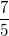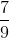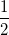Explanation:

A proprotion is the number of a specific event (blonde women) divided by the total number of events (total number of women).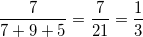Example Question #1 : Proportions And Percentages

Solve: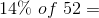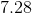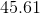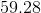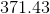Explanation: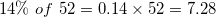Example Question #2 : Proportions And Percentages

Solve: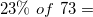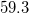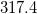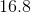Explanation: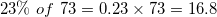Example Question #1 : Proportions And Percentages

A bag containsgreen marbles,red marbles, andblue marbles. What is the proportion of red marbles?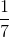Explanation:

The proportion is equal to the number of specific events (red marbles) divided by the total number of events (sum of all colors).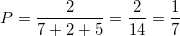Example Question #3 : Proportions And Percentages

What is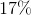of?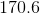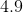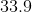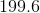Explanation: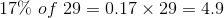Example Question #4 : Proportions And Percentages

Sherry deposits \$3,000 at 6% simple interest per year. How much will she have in this account after three years, assuming she does not make any withdrawals or deposits?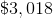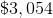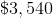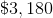Explanation:

Use the simple interest formula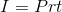with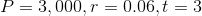: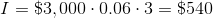This is the simple interest earned. Add it to the original principal: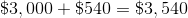Example Question #1 : Proportions And Percentages

The ratio of students to instructors at a prestigious law school is 15:4. If the school employs 120 instructors, then how many students total must it limit itself to enrolling in order to maintain this student-instructor ratio?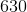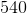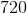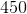Explanation:

Letbe the maximum number of students admitted.

For the school to maintain a 15:4 student-teacher ratio with 120 instructors, the ratios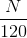and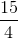must be equal. Therefore, we solve the proportion statement for: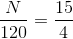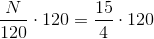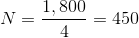The maximum number of students it can admit is 450.

Example Question #2 : Proportions And Percentages

The ratio of students to instructors at a prestigious medical school is 25:6. The school employs 90 instructors, and there will be 240 students returning. How many new students must it limit itself to admitting in order to continue to maintain this student-instructor ratio?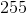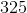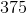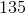Explanation:

Letbe the maximum number of students admitted overall.

For the school to maintain a 25:6 student-teacher ratio with 90 instructors, the ratios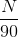and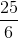must be equal. Therefore, we solve the proportion statement for: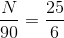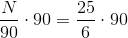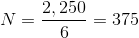Since 240 spots are already filled by returning students, the medical school can admit up to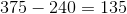new students.

Example Question #3 : Proportions And Percentages

Which of the following statements follows from the statement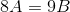?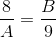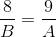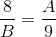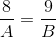Explanation:

Two ratios are equivalent if and only if their cross-products are equal. We look for the proportion statement whose cross-products are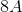and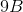. Of the four choices, onlyfits this criterion, so it is the correct choice.

Example Question #4 : Proportions And Percentages

Which of the following statements does not follow from the statement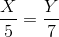?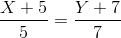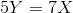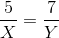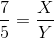Explanation:

Fromwe can conclude the following:is true, because the cross-products of a proportion statement are true.is true, because if two ratios are equivalent, their reciprocals are equivalent.can be demonstrated to be true as follows: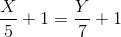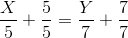The fourth statement is false: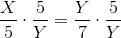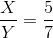, which contradicts the statement that.

← Previous 1 3 4 5 6 7 8 9